Journal of Electrical and Electronic Engineering
Volume 3, Issue 3, June 2015, Pages: 25-29

Analysis on the Basis of Volterra Series Signal–To–Noise Ratio of Nonlinear Device in the Conditions of the Stochastic Resonance Effect

1National Technical University "Kharkiv Polytechnic Institute», Kharkiv, Ukraine

2Scientific Center, Kharkiv Air Force University named I. Kozhedub, Kharkiv, Ukraine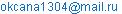(O. Kharchenko)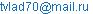(V. Tyutyunnik)

Okcana Kharchenko, Vladislav Tyutyunnik. Analysis on the Basis of Volterra Series Signal–To–Noise Ratio of Nonlinear Device in the Conditions of the Stochastic Resonance Effect. Journal of Electrical and Electronic Engineering. Vol. 3, No. 3, 2015, pp. 25-29.

Abstract: In this paper, the stochastic resonance effect is considered. It is shown that the stochastic resonance effect appears in the conditions of operating on the nonlinear system of additive mixture of desired signal and noise. The numerical simulation of the output signal when exposed to the input of the system of additive mixture harmonic signal and noise with a uniform distribution is given. Analytical relational expressions for signal-to-noise ratio on the output of the nonlinear system are got. The analysis of signal-to-noise ratio is conducted on the output of the nonlinear system depending on the parameters of the input signal and noise. In this paper we have shown the stochastic resonance effect occurs mainly at low frequencies.

Keywords: Stochastic Resonance (SR), Nonlinear System, Signal-to-Noise Ratio (SNR), Volterra Series

1. Introduction

In modern conditions the problem of providing of reliable communication of data at presence of interferences is considered one of major problems. Error-correcting codes, optimal filters are created, and are used by the detection method of accumulation, the probabilistic approach to suppressing random disturbances. etc. [1,2,3].

At the same time, researches by physicists at the end of the XX century , resulted in paradoxical conclusions. The noise on the input of the nonlinear systems possessing the effect of the so-called stochastic resonance (SR), allows to stand out a weak (as compared to the noise) signal from additive signal–noise mixture. The SR effect characterizes the response of the nonlinear system on a weak input signal. Thus data-output of the nonlinear system, such as an amplification factor and signal-to-noise ratio, at certain terms have the distinctly expressed maximum [4,5].

2. Concept of Stochastic Resonance

Stochastic resonance is a nonlinear physical phenomenon in which the output signals of some nonlinear systems can be enhanced by adding suitable noise under certain conditions [4,5]. SR is a universal effect, to many inherent nonlinear systems being under external influence simultaneously of chaotic and weak periodic influences. The response of the nonlinear system on a weak external signal in case of SR noticeably increases with the height of the noise intensity in the system and arrives at a certain maximum at some level.

Consider the nonlinear system described by the equation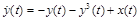(1)

where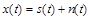is an input process being additive mixture of desired signal and normal noise;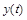is a process on the output of the nonlinear device.

As shown in  this system has the SR effect. The numeral simulation of response at affecting input of the system of additive mixture of harmonic signal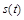and noise with even distribution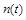, illustrating model (1), resulted on Fig.1.

It is seen that as a result of processing in accordance with expression (1) can significantly reduce the noise component fluctuations, although the form of the output signal differs from the harmonic. However, Equation (1) is an Abel equation of the second kind and has no exact analytical solution.

3. The Analysis of Nonlinear Systems using Volterra Series

The principle of superposition, which is the basis of the linear theory, is not applicable for the investigation of phenomena in nonlinear systems. Forced abandonment of the principle of superposition complicates research opportunities. Unfortunately, such powerful and versatile methods of research, which has the linear theory, is absent in the nonlinear theory. The study of nonlinear problems comprises a number of specialized techniques and methods that have different power and scope[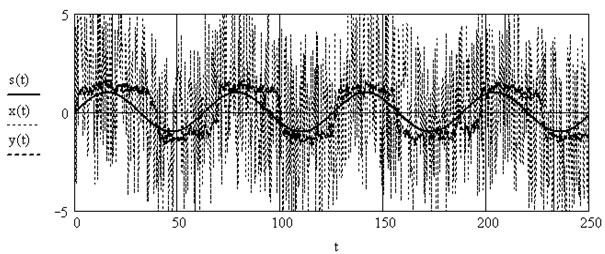Figure 1. Standing out of signal from additive signal–noise mixture.

To receive the signal at the output of a nonlinear system in the analytical form Volterra series are usually used. They are described as "power series with memory" for displaying an output signal of a nonlinear system in the form of degrees of the input signal . For the nonlinear system described equation (1) will use the following notation series.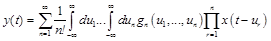(2)

whereis the output signal;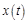is the input signal;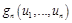are the n-th order Volterra kernels, which describe the system.

It should be noted that the kernel of the first order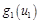is the pulse response of a linear circuit. Thus, the kernel of a higher order can be viewed as the pulse response of a high order, which are used to describe different orders of nonlinearity.

Appearing in the  coefficient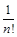introduced A. Bedrosian and D. Rice to simplify these expressions.

Important for in this analysis is n-order Fourier transform, which looks as follows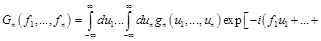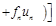(3)

Value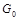equal to zero, as in the present case, the Volterra series begins with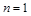. The member of the row with number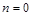corresponds to the active system (when there is an output signal when no input, which is contrary to the principle of causality).

Component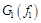is a transfer function of a linear circuit.

Thus, the transformation kernel of Volterra-th n-order is similar to the transfer function of the n-order. The complete formula of the form (2) are infinite series, and the complexity of computing the n-th member with increase of n increases rapidly. However, the analysis of communication systems by members of Volterra series above the second or third order can be neglected .

4. Calculation and Analysis of the Spectral Power Density of the Output Signal

Let us consider a nonlinear system described by equation (1). A harmonic signal is at the input of a nonlinear system. As shown in  this nonlinear system has the SR effect. Bedrosian A. and Rice D.  derived analytical expressions for the spectral power density of the components of the output signal of a nonlinear system using a series of Voltaire. The transfer functions for a given nonlinear system are defined in . The results of the calculations of the output power spectrum main members are given in table 1.

Here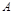is the amplitude of the harmonic input signal,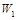is the spectral power of the noise component in the input signal. Further, to enhance the visibility and convenience of calculations in the resulting formula is used circular frequency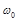instead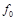.

If the input signal is an additive mixture of the unmodulated carrier and noise then the spectral power density of the output signal includes three main parts [9,10]: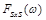corresponds to the beatings between signal components and its harmonics (discrete part of the spectrum);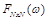corresponds to the beatings noise components (continuous part of the spectrum);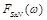corresponds to the beatings signal and noise components (continuous component of the spectrum).

Therefore, the energy spectrum of the output of the nonlinear system is defined as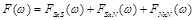(4)

In order to determine the signal-to-noise ratio (SNR) at the output of a nonlinear system, you must decide to signal or noise attributed to the part of the output spectrum. Thus, we get two expressions:

a) when the beating between the signal components and noise related to the noise: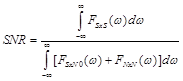(5)

b) when the beating between the signal components and noise related to the signal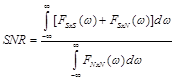(6)

In our case, the signal will include components of the first and third lines (Table 1). Other components refer to the output noise. The resulting formula for the signal-to-noise ratio is quite cumbersome, so we present the results of calculations in Fig. 2. The signal-to-noise ratio is a function of three variables:,and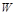.

Fig. 2. shows that SNR has a strongly pronounced maximum at a certain frequency at a given amplitude. Note that the SNR has a minimum too. In addition, the dependence of the SNR on the spectral power density of input noise is non-monotonic.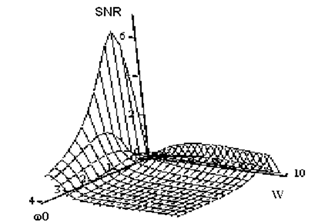Figure 2. SNR dependence on the frequency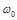of the input harmonic signal (A=1 – amplitude of input signal, W – spectral power density of input noise)

Table 1. The calculation results of the output power spectrum.

 The main members of the output power spectrum introduced by Bedrosian and Rrice The main members of the output power spectrum of a nonlinear system described by equation (1) The pulses due to the harmonic components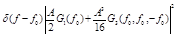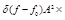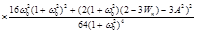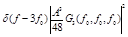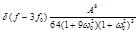Members of the negative frequency range when replacing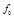for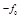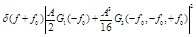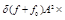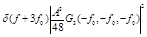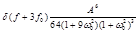Continuous part of the spectrum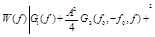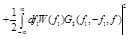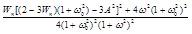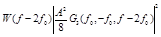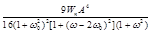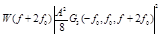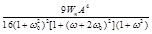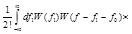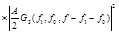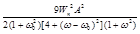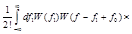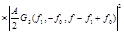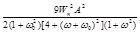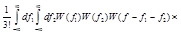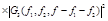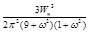As it is shown in Fig. 3, the SNR (for a given frequency of the first harmonic) is only a maximum value at certain value.

With increasing frequency of the harmonic signalthe SNR maximum is shifted in the direction of increasing of the spectral power density of input noise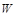. From the Figures it is seen that the SR effect is manifested mainly at low frequencies. Thus, at relatively low values of frequency using nonlinear equations (3) to solve the problem of the selection signal is at a high level of noise.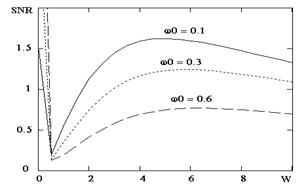Figure 3. The dependence of the SNR from the spectral power density of input noiseof the different values of frequency of the harmonic signal(the amplitude of the input sine signal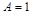)

5. Conclusion

In this paper, both by the numeral simulation and by the analytical form Volterra series simulation, the possibility of improving the output signal in the nonlinear system by adding noise has been established. An input process consisting of the additive mixture of the harmonic signal and the normal noise has been considered. Abel equation of the second kind in which an effect of stochastic resonance takes place is represented.

The analytical expressions for the components of the spectral power density of the nonlinear system output signal using Volterra series are deduced. Based on the method of decomposition in the Volterra series analysis of nonlinear equations describing the response of a nonlinear system, which has the SR effect.

Components of the power spectral density of the output signal of a nonlinear system are entered and calculated.

Signal-to-noise ratio at the output of the nonlinear device has been obtained and analyzed.

The values of the parameters of the input oscillations, corresponding to the SR effect have been shown. In this paper, we have established that the output signal amplitude increases with increasing noise intensity, passes through a maximum, and then decreases again.

It should be pointed out that the results obtained in this paper make it possible to calculate the optimum level of spectral power density of additive noise at which the SNR maximum is reached. One can see that the SNR dependence shows non-monotonic behavior with the increase of the spectral power density of input noiseand frequency of the harmonic signal.

It has been shown that by choosing the optimal level of the noise power, we can achieve the maximum level of the signal at a given frequency.

The analytical expressions deduced here can be the basis for the design of radio systems operating in conditions of high noise levels.

References

1. Kharkevich A.A. Borba s pomekhami [Interference Contro]l, M: Nauka, 1965, 275 p. (in Russian)
2. Sklar B. Digital Communication. Fundamentals and Applications, Second Edition, Prentice Hall PTR, 2003, 1099 p. (in Russian)
3. Tikhonov V.I. Statisticheskay rediotechnika [Statistical Radioengineering], M: Sov. Radio, 1966, 678 p. (in Russian)
4. Anishenko V.S., Neman V.B., F., Gayer L. Stokhasticheskiy resonans kak indyshirovanay shumom effect uvelicheniy stepeni poraydka. [Stochastic resonance as induced by noise the effect of increasing the degree of order]. – Uspekhi fizicheskikh nayk, 1999, vol. 169, № 1, pp. 7–37. (in Russian)
5. Kharchenko O.I., Chumakov V.I., Sklyaruk V.L. K raschotu otklika nelineynoi sistemy s pemyatu s primeneniem rjaydov Volterra. [On the calculation response of the nonlinear system with memory response using the Voltaire series]. Zb. Nauk. prats AMVS,2010, vip. 3(3), pp. 110-115. . (in Russian
6. Geraschenko O.V. Stokhasticheskiy resonans v asimmetrichnoi bistabilnoy sisteme. [Stochastic resonance in an asymmetric bistable system]. Pisma v GTF, 2003, vol. 29, vyp. 6, pp.82-86. (in Russian)
7. Kharkevich A.A. Nelineynye i parametricheskiy ustroyctva. [Nonlinear and parametric devices]. M.: Gosudarstvenoe izd. tekhniko-teoreticheskoi literatury, 1955, 184 p. (in Russian)
8. Bedrosian E., Rice S. The Output Properties of Volterra Systems (Nonlinear Systems with Memory) Driven by Harmonic and Gaussian Inputs // IEEE.- Vol. 59, No 12.- 1971. – pp.58–82.
9. Levin B.R. Teoreticheskie osnovy statisticheskoi radiotekhniki. [Theoretical foundations of statistical radio engineering]. M: Sov. Radio, 1969, 752 p. (in Russian)
10. David Middleton, An Introduction to Statistical Communication Theory: An IEEE Press Classic Reissue, Wiley-IEEE Press, 1996.–1184 p. (in Russian)

 Contents 1. 2. 3. 4. 5.
Article ToolsAbstractPDF(2643K)